PSABPQXA - Student Facing Task---Acc7.3 Lesson 3 Reasoning about Equations with Tape Diagrams (6.EE.B.5, 7.EE.B.3, 7.EE.B.4.a)
Part A)

3.1: Find Equivalent Expressions

Select all the expressions that are equivalent to 7(2 - 3n) . Explain how you know each expression you select is equivalent.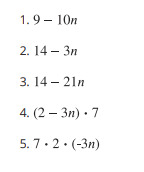Select all that apply:
Part B)

Explain how you know each expression you select is equivalent.

Part A)

3.2: Matching Equations to Tape Diagrams

1. Match each equation to one of the tape diagrams. Be prepared to explain how the equation matches the diagram.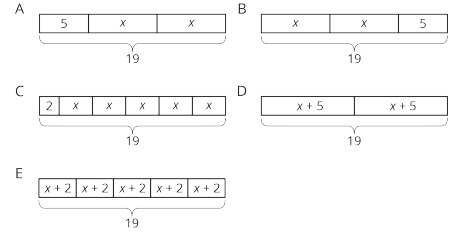2x + 5 = 19 matches with which tape diagram?

Select one:
Part B)

3.2: Matching Equations to Tape Diagrams

1. Match each equation to one of the tape diagrams. Be prepared to explain how the equation matches the diagram.2 + 5x = 19 matches with which tape diagram?

Select one:
Part C)

3.2: Matching Equations to Tape Diagrams

1. Match each equation to one of the tape diagrams. Be prepared to explain how the equation matches the diagram.2(x + 5) = 19 matches with which tape diagram?

Type your answer below as a number (example: 5, 3.1, 4 1/2, or 3/2):
Part D)

3.2: Matching Equations to Tape Diagrams

1. Match each equation to one of the tape diagrams. Be prepared to explain how the equation matches the diagram.5(x + 2) = 19 matches with which tape diagram?

Select one:
Part E)

3.2: Matching Equations to Tape Diagrams

1. Match each equation to one of the tape diagrams. Be prepared to explain how the equation matches the diagram.19 = 5 + 2x matches with which tape diagram?

Type your answer below as a number (example: 5, 3.1, 4 1/2, or 3/2):
Part F)

3.2: Matching Equations to Tape Diagrams

1. Match each equation to one of the tape diagrams. Be prepared to explain how the equation matches the diagram.(x + 5)2 = 19 matches with which tape diagram?

Type your answer below as a number (example: 5, 3.1, 4 1/2, or 3/2):
Part G)

3.2: Matching Equations to Tape Diagrams

1. Match each equation to one of the tape diagrams. Be prepared to explain how the equation matches the diagram.19 = (x + 2)5 matches with which tape diagram?

Type your answer below as a number (example: 5, 3.1, 4 1/2, or 3/2):
Part H)

3.2: Matching Equations to Tape Diagrams

1. Match each equation to one of the tape diagrams. Be prepared to explain how the equation matches the diagram.192 = x + 5 matches with which tape diagram?

Select one:
Part I)

3.2: Matching Equations to Tape Diagrams

1. Match each equation to one of the tape diagrams. Be prepared to explain how the equation matches the diagram.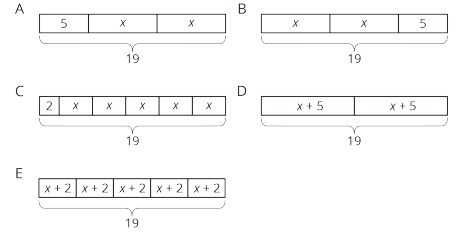19 - 2 = 5x matches with which tape diagram?

Select one:
Part J)

2. Sort the equations into categories of your choosing. Explain the criteria for each category.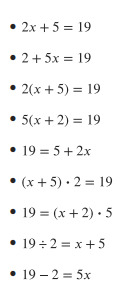Part A)

3.3: Drawing Tape Diagrams to Represent Equations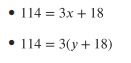Draw a tape diagram to match each equation.

Upload a picture of your tape diagrams using the "insert image" icon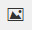. If you can't upload an image, describe in detail what your tape diagrams look like.

Part B)

Use any method to find values for x and y that make the equations true.Type your answer below as a number (example: 5, 3.1, 4 1/2, or 3/2):
Part C)
Type your answer below as a number (example: 5, 3.1, 4 1/2, or 3/2):
Part A)

To make a Koch snowflake: Start with an equilateral triangle that has side lengths of 1. This is step 1.

Replace the middle third of each line segment with a small equilateral triangle with the middle third of the segment forming the base. This is step 2.

Do the same to each of the line segments. This is step 3.

Keep repeating this process.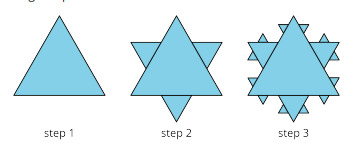What is the perimeter after step 2?

Type your answer below as a number (example: 5, 3.1, 4 1/2, or 3/2):
Part B)

What is the perimeter after step 3?

Don't use labels (units) in your answer. This answer should be an improper fraction with the larger number on top.